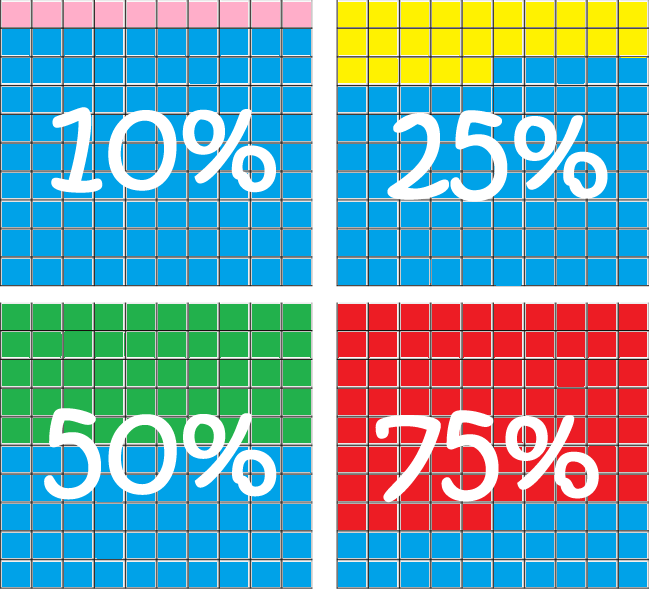# Definition of PercentageA Percentage is a number that gives you a fraction of $100$.

We use the symbol $\%$ for percentages.

A percentage gives you the numerator of a fraction with a denominator of $100$.

For example,

• $10\% = \dfrac{10}{100}$
• $25\% = \dfrac{25}{100}= \dfrac{1}{4}$
• $50\% = \dfrac{50}{100} = \dfrac{1}{2}$
• $75 \% = \dfrac{75}{100} = \dfrac{3}{4}$
• $100 \% = \dfrac{100}{100} = 1$

### Description

The aim of this dictionary is to provide definitions to common mathematical terms. Students learn a new math skill every week at school, sometimes just before they start a new skill, if they want to look at what a specific term means, this is where this dictionary will become handy and a go-to guide for a student.

### Audience

Year 1 to Year 12 students

### Learning Objectives

Learn common math terms starting with letter P

Author: Subject Coach
Added on: 6th Feb 2018

You must be logged in as Student to ask a Question.

None just yet!